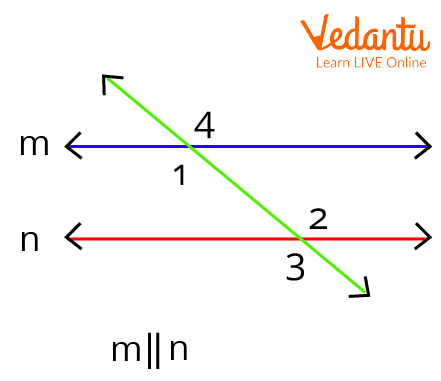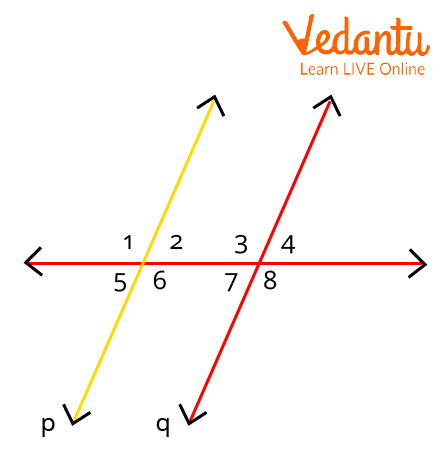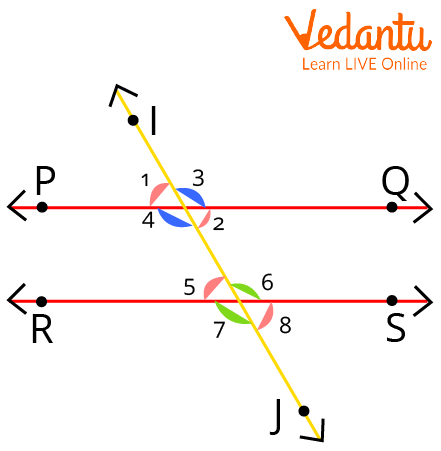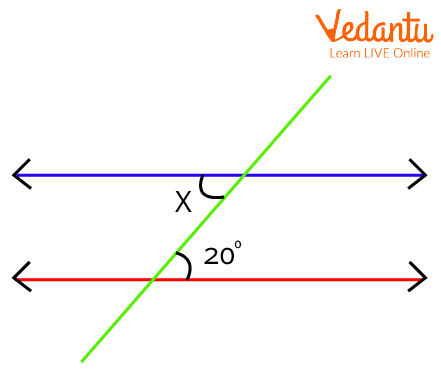Courses
Courses for Kids
Free study material
Offline Centres
More

# Corresponding Angle and Alternate Angle TheoremsLast updated date: 29th Nov 2023
Total views: 121.5k
Views today: 2.21k## An Introduction to Corresponding and Alternate Angle Theorems

Corresponding and Alternate angles are the most fundamental angles formed when two parallel lines are intersected by a transversal line. In this article, we will discuss the Corresponding and Alternate Angle Theorems in detail along with their proof in detail. Corresponding and Alternate Angles are the most widely used tool in Geometry. Parallel lines find many applications in our day-to-day life and so do the properties of parallel lines such as corresponding and alternate angles. Corresponding and Alternate Angles theorems form a fundamental tool of Euclidean Geometry.

## Statement of Corresponding and Alternate Angle Theorems

### Alternate Angle Theorem

The theorem states that if a line referred to as transversal intersects parallel lines, the alternate interior angles are congruent.

### Corresponding Angle Theorem

The theorem states that if a transversal intersects parallel lines, the corresponding angles are congruent.Angles made by two parallel lines m and n

In the above diagram, these $\angle 1=\angle 3, \angle 2=\angle 4$ are the corresponding angles, and $\angle 1=\angle 2$ are alternate interior angles.

## Proof of Corresponding and Alternate Theorems

### Alternate Angles Theorem

Given: Lines $p\parallel q$

To prove: $\angle 2 = \angle 7$ and $\angle 3 = \angle 6$Proof of Alternate Angle Theorem

Proof: Let us assume that $p$ and $q$ are two parallel lines and $t$ is the transversal that intersects parallel lines $p$ and $q$. We know that if a transversal intersects any two parallel lines, the corresponding angles and vertically opposite angles are equal.

Therefore,

$\Rightarrow \angle 1 = \angle 3 \quad \ldots$ (i) (Corresponding angles)

$\Rightarrow \angle 1 = \angle 6$....(ii) (Vertically opposite angles)

From equations (i) and (ii), we get

$\Rightarrow \angle 3 = \angle 6 \quad$ (Alternate interior angles)

Similarly,

$\Rightarrow \angle 2 = \angle 7$

Hence, the proof of the Alternate Angle Theorem.

### Corresponding Angles TheoremProof of Corresponding angle Theorem

To Prove:

$\angle 1 = \angle 5,\angle 3 = \angle 6,\angle 4 = \angle 7,\angle 2 = \angle 8$

Given, PQ and RS are the two parallel lines intersected by the transversal IJ.

Now, if $PQ\parallel RS$, then by the corresponding angles theorem, we can write

$\angle 1 = \angle 5$

$\angle 3 = \angle 6$

$\angle 4 = \angle 7$

$\angle 2 = \angle 8$

Remember: The theorem can only be proved when parallel lines are given.

Thus, this theorem is true without proof.

## Limitations of Corresponding and Alternate Angle Theorems

• Both the theorems are applicable only if transversal lines intersect the parallel lines.

• They are not applicable in the case of nonparallel lines as the nonparallel lines do not form corresponding and alternate angles.

## Applications of Corresponding and Alternate Angle Theorems

• Both Theorems are applicable in geometry to solve questions related to corresponding angles and alternate angles.

• Rubik's Cube, railway tracks, and opening and shutting of geometry boxes are examples of corresponding angles.

## Solved Examples

1. Find the value of angle $x$ in the given figure if the two lines are parallel and they are crossed by a transversal.Given figure to find angle x

Ans:

By the alternate interior angles theorem,

$x$ and $20^{\circ}$ are the alternate interior angles. Hence, they are equal.

Therefore,

$\Rightarrow x = 20^{\circ}$.

2. The two corresponding angles are given to be $9 x+10$ and 64. What is the value of $x$?

Ans: The two given corresponding angles are congruent.

$9 x+10= 64 \\$

$\Rightarrow 9 x=64-10 \\$

$\Rightarrow 9 x=54 \\$

$\Rightarrow x=6$

3. The values of two corresponding angles $\angle 2=5 x+6$ and $\angle 6=3 x+18$. Solve for the value of $x$.

Ans: As they are corresponding angles and the lines are said to be parallel in nature, then they should be congruent.

Equate the given expressions $\angle 2=5 x+2$ and $\angle 6=3 x+10$ and find the value of $x$.

$5 x+6=3 x+18$

$\Rightarrow 5 x-3 x=18-6$

$\Rightarrow 2 x=12$

$\Rightarrow x=\dfrac{12}{2}$

$\Rightarrow x=6$

## Important Formulas to Remember

• Corresponding angles theorem: If two parallel lines are intersected by transversal lines, then the corresponding angles are equal.

• Alternate angles theorem: If two parallel lines are intersected by transversal lines, then alternate angles are equal.

## Important Points to Remember

• Corresponding Angles are formed on the same side of parallel lines intersected by a transversal line.

• Alternate angles are formed on opposite sides of parallel lines intersected by a transversal line.

## Conclusion

In the article, we have discussed the detailed proof of Corresponding and Alternate Angle Theorems and the applications of the corresponding and alternate angles. These theorems are fundamental tools in geometry and form the basis of angle theory. These theorems are of great importance and so are necessary to be studied.

## FAQs on Corresponding Angle and Alternate Angle Theorems

1. What is meant by corresponding angles?

Corresponding angles are the angles that are formed when two parallel lines are interested in a line known as transversal. Corresponding angles are those angles that occupy the same relative position at each of the intersections when two parallel lines are cut by a third line which is referred to as a transversal line. We have a theorem for corresponding angles which is known as the corresponding angle theorem. The converse of the Corresponding Angle theorem is also true, i.e., if Corresponding angles formed between two lines cut by a third line are equal, then the lines must be parallel lines.

2. What is meant by alternate angles?

Alternate angles are the angles that are formed when two parallel lines are cut by a transversal. Alternate angles are the angles that are formed on the alternate sides of the transversal. These angles do not have the same vertices. There are two types of alternate angles.

• Alternate interior angles

• Alternate exterior angles

The alternate angles which are formed within the intersection region are said to be alternate interior angles and the alternate angles which are formed on the exterior side of the intersection region are called alternate exterior angles.

3. What is the difference between corresponding and alternate angles?

Both the alternate and corresponding angles are formed when two parallel lines are cut by a transversal. But both types of angles are different from each other.

• Alternate angles are formed on the opposite side of the transversal which cuts the parallel lines; on the other hand, the corresponding angles are on the same side of the transversal.

• Alternate angles are either both inside the parallel lines, i.e., alternate interior angles, or both outside the parallel lines, i.e., alternate exterior angles. On the other hand, the corresponding angles are always one inside the parallel lines and the other outside the parallel lines.